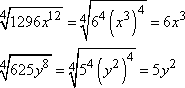Search

The Binomial Theorem: Examples (page 2 of 2)

Sections: The formulas, Worked examples• Expand (x2 + 3)6
• Students trying to do this expansion in their heads tend to mess up the powers. But this isn't the time to worry about that square on the x. I need to start my answer by plugging the terms and power into the Theorem. The first term in the binomial is "x2", the second term in "3", and the power n is 6, so, counting from 0 to 6, the Binomial Theorem gives me:

(x2 + 3)6  =  6C0 (x2)6(3)0 + 6C1 (x2)5(3)1 + 6C2 (x2)4(3)2 + 6C3 (x2)3(3)3

+ 6C4 (x2)2(3)4 + 6C5 (x2)1(3)5 + 6C6 (x2)0(3)6

Then simplifying gives me

(1)(x12)(1) + (6)(x10)(3) + (15)(x8)(9) + (20)(x6)(27)

+ (15)(x4)(81) + (6)(x2)(243) + (1)(1)(729)

= x12 + 18x10 + 135x8 + 540x6 + 1215x4 + 1458x2 + 729

• Expand (2x  5y)7
• I'll plug "2x", "5y", and "7" into the Binomial Theorem, counting up from zero to seven to get each term. (I mustn't forget the "minus" sign that goes with the second term in the binomial.)

(2x  5y)7 = 7C0 (2x)7(5y)0 + 7C1 (2x)6(5y)1 + 7C2 (2x)5(5y)2

+ 7C3 (2x)4(5y)3 + 7C4 (2x)3(5y)4 + 7C5 (2x)2(5y)5

+ 7C6 (2x)1(5y)6 + 7C7 (2x)0(5y)7

Then simplifying gives me:   Copyright © Elizabeth Stapel 1999-2009 All Rights Reserved

(1)(128x7)(1) + (7)(64x6)(5y) + (21)(32x5)(25y2) + (35)(16x4)(125y3)

+ (35)(8x3)(625y4) + (21)(4x2)(3125y5) + (7)(2x)(15625y6)

+ (1)(1)(78125y7)

= 128x7  2240x6y + 16800x5y2  70000x4y3 + 175000x3y4  262500x2y5

+ 218750xy6  78125y7

You may be asked to find a certain term in an expansion, the idea being that the exercise will be way easy if you've memorized the Theorem, but will be difficult or impossible if you haven't. So memorize the Theorem and get the easy points.

• What is the fourth term in the expansion of (3x  2)10?
• I've already expanded this binomial, so let's take a look:

(3x  2)10 = 10C0 (3x)100(2)0 + 10C1 (3x)101(2)1 + 10C2 (3x)102(2)2

+ 10C3 (3x)103(2)3 + 10C4 (3x)104(2)4 + 10C5 (3x)105(2)5

+ 10C6 (3x)106(2)6 + 10C7 (3x)107(2)7 + 10C8 (3x)108(2)8

+ 10C9 (3x)109(2)9 + 10C10 (3x)1010(2)10

So the fourth term is not the one where I've counted up to 4, but the one where I've counted up just to 3. (This is because, just as with Javascript, the counting starts with 0, not 1.)

Note that, in any expansion, there is one more term than the number in the power. For instance:

(x + y)2 = x2 + 2xy + y2   (second power: three terms)

(x + y)3 = x3 + 3x2y + 3xy2 + y3   (third power: four terms)

(x + y)4 = x4 + 4x3y + 6x2y2 + 4xy3 + y4   (fourth power: five terms)

The expansion in this exercise, (3x  2)10, has power of n = 10, so the expansion will have eleven terms, and the terms will count up, not from 1 to 10 or from 1 to 11, but from 0 to 10. This is why the fourth term will not the one where I'm using "4" as my counter, but will be the one where I'm using "3".

10C3 (3x)103(2)3 = (120)(2187)(x7)(8) = 2099520x7

• Find the tenth term in the expansion of (x + 3)12.
• To find the tenth term, I plug x, 3, and 12 into the Binomial Theorem, using the number 10  1 = 9 as my counter:

12C9 (x)129(3)9 = (220)x3(19683) = 4330260x3

• Find the middle term in the expansion of (4x  y)8.
• Since this binomial is to the power 8, there will be nine terms in the expansion, which makes the fifth term the middle one. So I'll plug 4x, y, and 8 into the Binomial Theorem, using the number 5  1 = 4 as my counter.

8C4 (4x)84y)4 = (70)(256x4)(y4) = 17920x4y4

You might be asked to work backwards.

• Express 1296x12  4320x9y2 + 5400x6y4  3000x3y6 + 625y8 in the form (a + b)n.
• I know that the first term is of the form an, because, for whatever n is, the first term is nC0 (which always equals 1) times an times b0 (which also equals 1). So 1296x12 = an. By the same reasoning, the last term is bn, so 625y8 = bn. And since there are alternating "plus" and "minus" signs, I know from experience that the sign in the middle has to be a "minus". (If all the signs had been "plusses", then the middle sign would have been a "plus" also. But in this case, I'm really looking for "(a  b)n".)

I know that, for any power n, the expansion has n + 1 terms. Since this has 5 terms, this tells me that n = 4. So to find a and b, I only have to take the 4th root of the first and last terms of the expanded polynomial:Then a = 6x3, b = 5y2, there is a "minus" sign in the middle, and:

1296x12  4320x9y2 + 5400x6y4  3000x3y6 + 625y8 = (6x3  5y2)4

Don't let the Binomial Theorem scare you. It's just another formula to memorize. A really complicated and annoying formula, I'll grant you, but just a formula, nonetheless. Don't overthink the Theorem; there is nothing deep or meaningful here. Just memorize it, and move on.

<< Previous  Top  |  1 | 2  |  Return to Index

 Cite this article as: Stapel, Elizabeth. "The Binomial Theorem: Examples." Purplemath. Available from     https://www.purplemath.com/modules/binomial2.htm. Accessed [Date] [Month] 2016

Study Skills Survey

Tutoring from Purplemath
Find a local math tutor

 Copyright © 2021  Elizabeth Stapel   |   About   |   Terms of Use   |   Linking   |   Site Licensing Contact Us